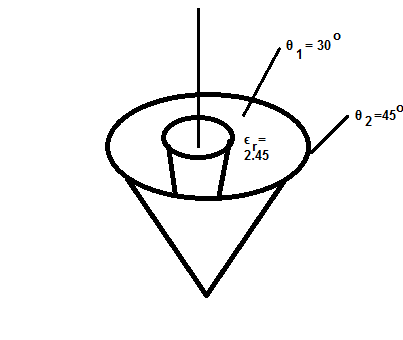In the figure the cone at theta = 45 degree has a voltage V with respect to the reference at...

Question:

In the figure the cone at {eq}\theta = 45 ^\circ {/eq} has a voltage V with respect to the reference at {eq}\theta = 30 ^\circ {/eq}. At {eq}r = 0.25\ m {/eq} and {eq}\theta = 30 ^\circ {/eq}, {eq}E = 2.30 \times 10^3 a_0\ \dfrac V m {/eq}. Determine the voltage difference V.Voltage:

It can be defined as the capacity of the system that can be utilized when a charged particle-induced from an infinite point to a given point. In the parallel circuit, it remains the same throughout the process.

Become a Study.com member to unlock this answer! Create your account

Given Data:

• The radius of a cone is, {eq}{r_1} = 0.25\;{\rm{m}} {/eq}
• The electric field is, {eq}E = 2.30 \times {0^3}{a_0}\;{\rm{V/m}} {/eq}

T...Voltage Sources: Energy Conversion and Examples

from

Chapter 6 / Lesson 6
21K

Electrons are motivated to power circuits through voltage sources, which can be clearly seen in batteries and other everyday items. Generators can come in the form of nuclear, coal, natural gas, hydroelectric, and wind power to generate voltage. In this lesson, learn about energy conversion, voltage, voltage sources, and examples of these things in everyday life, including batteries and generators.